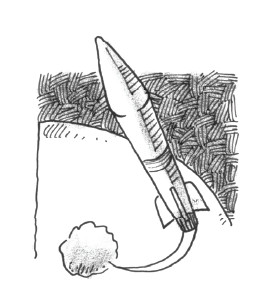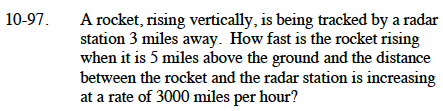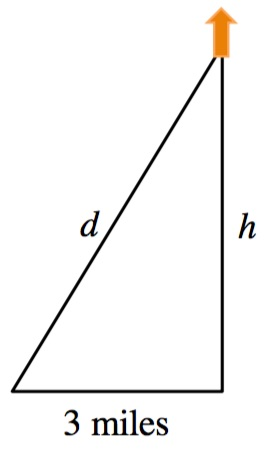### Home > CALC > Chapter 10 > Lesson 10.2.1 > Problem10-97

10-97.

A rocket, rising vertically, is being tracked by a radar station 3 miles away. How fast is the rocket rising when it is 5miles above the ground and the distance between the rocket and the radar station is increasing at a rate of 3000 miles per hour? Homework Help ✎Draw a diagram and use it to write an equation.Differentiate your equation from Step 1.

What is the value of d when h = 5?

Evaluate your equation from Step 2 for h = 5, d = (# from Step 3), and d′ = 3000.